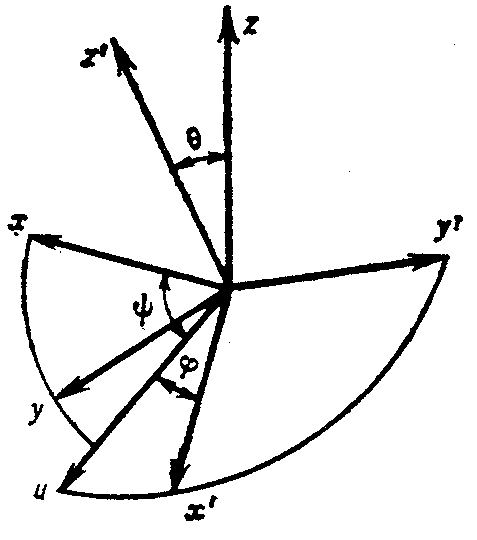# Euler angles

The angles $\phi$, $\psi$ and $\theta$ that determine the position of one Cartesian rectangular coordinate system $0xyz$ relative to another one $0x'y'z'$ with the same origin and orientation. The Euler angles are regarded as the angles through which the former must be successively rotated about the axes of the latter so that in the end the two systems coincide (see Fig.).Figure: e036390a

Let $u$ be the axis coinciding with the line of intersection of the planes $0xy$ and $0x'y'$, oriented so that the three lines $0z$, $0z'$ and $u$ form a right-handed triple. Then $\psi$ is the angle between $0x$ and $u$, measured in the plane $0xy$ from $0x$ in the direction of the shortest rotation of $0x$ to $0y$, $\theta$ is the angle between $0z$ and $0z'$ not exceeding $\pi$, and $\phi$ is in the direction of the shortest rotation of $0x'$ to $0y'$. The coordinates $x,y,z$, and $x',y',z'$ are connected by the relations

$$x'=(\cos\psi\cos\phi-\sin\psi\cos\theta\sin\phi)x'+$$

$$+(-\cos\psi\sin\phi-\sin\psi\cos\theta\cos\phi)y'+(\sin\psi\sin\theta)z',$$

$$y=(\sin\psi\cos\phi+\cos\psi\cos\theta\sin\phi)x'+$$

$$+(-\sin\psi\sin\phi+\cos\psi\cos\theta\cos\phi)y'+(-\cos\psi\sin\theta)z',$$

$$z=(\sin\theta\sin\phi)x'+(\sin\theta\cos\phi)y'+(\cos\theta)z'.$$

These angles were introduced by L. Euler (1748).